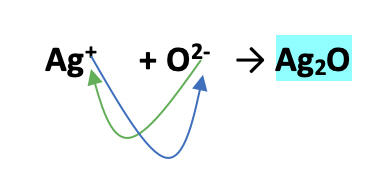# Problem: You may want to reference (Pages 88 - 90)Section 3.2 while completing this problem.Which of the following reactions is the balanced equation that represents the decomposition reaction that occurs when silver (I) oxide is heated?(a) Ag2O(s) →2Ag(s) + O(g)(b) Ag2O(s) →2Ag(s) + O2(g) (c) 2Ag2O(s) → 4Ag(s) + O2(g)(d) 2AgO(s) → 2Ag(s) +O2(g)(e) AgO(s) → Ag(s) + O(g)

###### FREE Expert Solution

silver(I) oxide###### Problem Details

You may want to reference (Pages 88 - 90)Section 3.2 while completing this problem.

Which of the following reactions is the balanced equation that represents the decomposition reaction that occurs when silver (I) oxide is heated?

(a) Ag2O(s) →2Ag(s) + O(g)

(b) Ag2O(s) →2Ag(s) + O2(g)

(c) 2Ag2O(s) → 4Ag(s) + O2(g)

(d) 2AgO(s) → 2Ag(s) +O2(g)

(e) AgO(s) → Ag(s) + O(g)